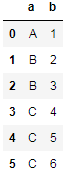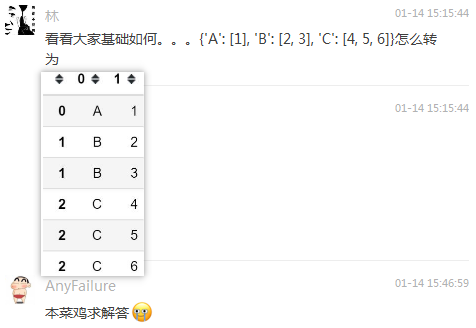## 基础解法explode函数

``````import pandas as pd

mydict = {'A': , 'B': [2, 3], 'C': [4, 5, 6]}
pd.DataFrame(mydict.items()).explode(1)
``````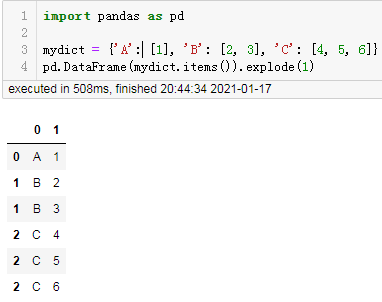`mydict.items()`是python基础字典的内容，它返回了这个字典键值对组成的元组列表：

``````mydict.items()
``````

``````dict_items([('A', ), ('B', [2, 3]), ('C', [4, 5, 6])])
``````

``````pd.DataFrame(mydict.items())
``````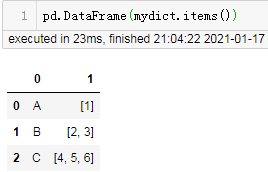``````select a,b_i from df lateral view explode(b) tmp as b_i;
``````

## 没有exlode函数如何解决这个问题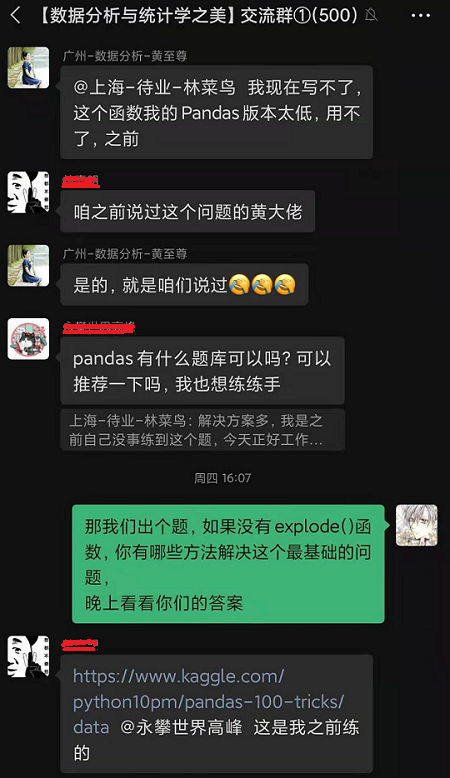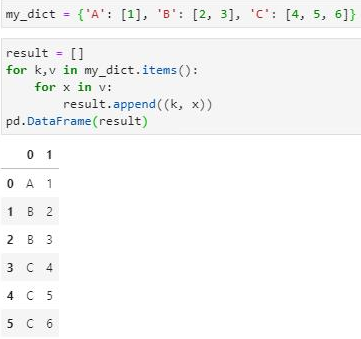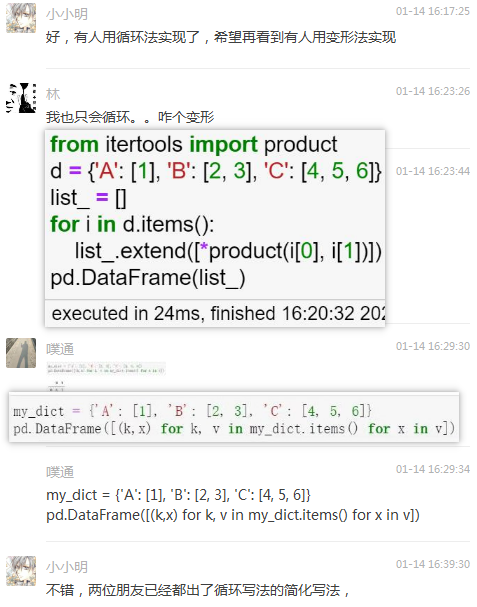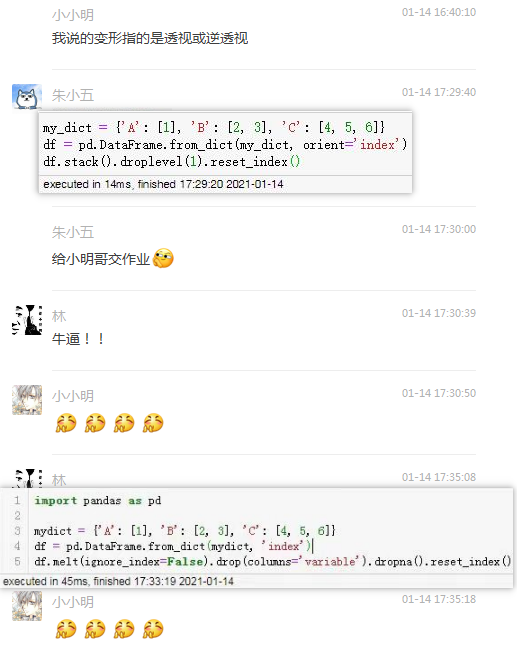## 循环法解题

``````result = []
for k, vs in mydict.items():
for v in vs:
result.append((k, v))
pd.DataFrame(result)
``````

``````import itertools
result = []
for k, v in mydict.items():
result.extend(itertools.product(k, v))
pd.DataFrame(result)
``````

``````product(*iterables, repeat=1) --> product object
``````

• iterables 为可迭代对象
• 可选参数repeat 表示重复次数

• product(‘ab’, range(3)) --> (‘a’,0) (‘a’,1) (‘a’,2) (‘b’,0) (‘b’,1) (‘b’,2)
• product((0,1), (0,1), (0,1)) --> (0,0,0) (0,0,1) (0,1,0) (0,1,1) (1,0,0) …

``````result = [(k, v) for k, vs in mydict.items() for v in vs]
pd.DataFrame(result)
``````

## 变形法解题

``````df = pd.DataFrame(mydict.items(), columns=["a", "b"])
df
``````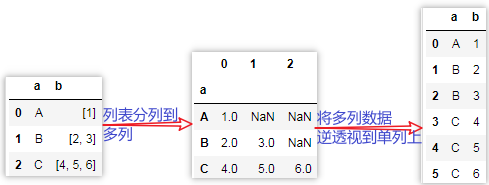### 列表分列的2种方法

``````df["b"].apply(pd.Series)
``````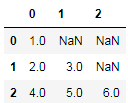``````df.set_index("a")["b"].apply(pd.Series)
``````

``````df["b"].apply(pd.Series).set_index(df["a"])
``````

``````df.agg({"a": lambda x: x, "b": pd.Series})
``````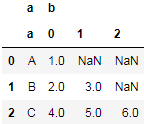``````df.agg({"a": lambda x: x, "b": pd.Series}).droplevel(0, axis=1)
``````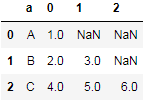``````df.agg({"a": lambda x: x, "b": pd.Series}).droplevel(0, axis=1).set_index("a")
``````

### 将字典的键作为索引的2种读取方法

``````df = pd.DataFrame.from_dict(mydict, 'index')
``````

``````df = pd.DataFrame(data=mydict.values(), index=mydict.keys())
``````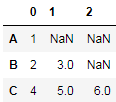### melt实现逆透视

``````df = df.rename_axis(index="a").reset_index()
df
``````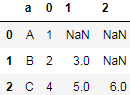``````df.melt(id_vars='a', value_name='b')
``````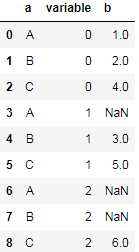``````df = pd.DataFrame.from_dict(mydict, 'index')
df = df.melt(id_vars='a', value_name='b').drop(columns="variable").dropna()
df.b = df.b.astype("int")
df
``````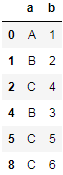### stack实现逆透视

``````df = pd.DataFrame.from_dict(mydict, 'index')
df.stack()
``````

``````A  0    1.0
B  0    2.0
1    3.0
C  0    4.0
1    5.0
2    6.0
dtype: float64
``````

``````df.stack().droplevel(1)
``````

``````A    1.0
B    2.0
B    3.0
C    4.0
C    5.0
C    6.0
dtype: float64
``````

``````df.stack().droplevel(1).reset_index()
``````

``````df.stack().droplevel(1).reset_index().set_axis(["a", "b"], axis=1)
``````

``````df.b = df.b.astype("int")
``````

``````df = pd.DataFrame.from_dict(mydict, 'index')
df = df.stack().droplevel(1).reset_index().set_axis(["a", "b"], axis=1)
df.b = df.b.astype("int")
df
``````